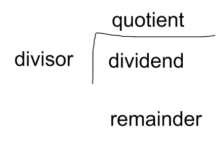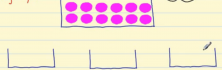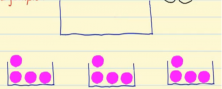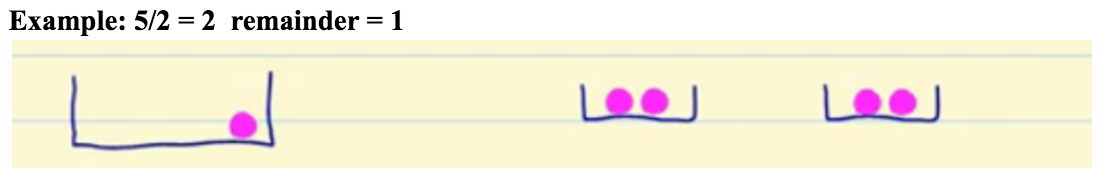Smartick is a fun way to learn math!Sep20

# Practice Dividing with and without Remainders

Dividing is equitable distribution, i.e. splitting between equal parts or groups.

### What is division?• Dividend: The number that you have to divide.
• Divisor: The number that the dividend is being divided by
• Quotient: The result of the division.
• Remainder: What is left over after the division

### Dividing with No Remainders

We want to distribute these 12 balls into 3 boxes in equal parts, therefore there must be the same number of balls in each of the boxes.Let’s start distributing the balls in each box until finally there are 4 balls in each box.So the division we have done is 12 balls / 3 boxes = 4 balls in each box.

Division is an inverse operation to multiplication and can be considered as repeated subtraction.

For example if we divide 12 by 3:
12 – 3 = 9
9 – 3 = 6
6 – 3 = 3
3 – 3 = 0

The number of times we can subtract 3 from 12 is 4, so 12/3 = 4.

### Dividing with Remainders or Inexact Divisions

The remainder is the amount left over after dividing one number by another.
For example: if we divide 5 balls between 2 boxes, we have two balls in each box and we are left with one, so 5/ 2 = 2 and the remainder is 1.In Smartick you can find many division problems with or without remainders. Join in and practice elementary math in a fun way!

Fun is our brain’s favorite way of learning
Diane Ackerman
Smartick is a fun way to learn math
• 15 fun minutes a day